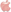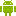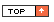Online Mathematics Resourcesindex

Useful Sites for Mathematics
Age Calculator (at Maths Cats)
BBC Schools - Numeracy
BBC Skillswise - Practical MathsBBC Teach - Maths (Ages 5-7)BBC Teach - Maths (Ages 7-11)Cloud Click
Espresso Fractions (Upper KS2)
Estimation 180
Estimation 2.02
Figure This!
Fresh Baked Fractions
Illustrated Maths Dictionary
Irregular Shapes Interactice Tutorial
Long Division Tool
Magic Math Market
Math Antics (Free Video Lessons)Math Cats
Math Dictionary
Math Glossary of Terms
MathigonMath Live (Real Life Math Applications)
Math Playground
Maths Championships (at Espresso)
Maths Dictionary for Kids
Mental Strategies for Addition and SubtractionPrimary Games (Software Samples)
Problem Solving Brain Teasers
Puzzle Playground
Rainforest Maths
R�omhfhocl�ir Matamaitice (ag CCEA)RobocompassSolve Me Puzzles (Pre-Algebra)

Maths IWB Resources
Abacus
Angle Measurer (at TeacherLed.com)Angle Measuring Tool (at TeacherLed.com)
Angle Sums (at Illuminations)
Area Tool - Advanced (at Illuminations) (Use IE)
Base Block Subtraction
Base 10 Blocks
Base 10 Manipulatives
Basic Angle Tool
Big Blue Calculator
Clock (ag Toy Theater)An Clog Cainteach (ag CCEA)
Co-ordinates Grid (Use IE)
Counting Fish
Crick's Interactive 100 Square
Equivalence Number Line
Equivalence Teaching Tool - Bars
Equivalence Teaching Tool - Pies
Equivalence Teaching Tool - Pies and Bars
Equivalent Fractions Demonstration Tool (at ICT Games)Equivalent Fractions Teaching Tool (at Illuminations)Fish Times Tables
Fraction Bars (at Math Playground)
Fraction Bars (at Teacher Led.Com)
Fraction Paint 2x2
Fraction Paint 3x3
Fraction Paint 4x4
Fraction Paint 5x5
Fraction Paint 6x6
Fractions Teaching Tool (at Math Learning Center)Fraction Scale (at Math Playground)
Fraction Wall
Geoboard (at Math Learning Center)Geoboard (at Math Playground)Geoboard (at NL Virtual Manipulatives)
Geoboard (at Toy Theater)Geometric Solids (at Illuminations)
Grid Maths
Interactive Circle (at Learn Alberta)
Interactive Clock (Time, Angles and Fractions)
Interactive 100 Number Chart (at ABCYa) (Use IE)
Interactive 100 Square (at ICT Games)Interactive Hundreds Chart (at Math Playground)Interactive Spinners (To Investigate Chance and Probability) (Use IE)
Interactive Triangles
Money Machine (at Teaching Money)
Multiple Representations Tool (at TT Rock Stars)Number Line (at Math Learning Center)Number Line Interactive
Number Frames (at Math Learning Center)Number Pieces (at Math Learning Center)Number Rack (at Math Learning Center)Pattern Shapes (at Math Learning Center)Percentage Fraction Decimals Grid
Percentage Fraction Decimal Wheel
Percentage Paint
Pictoram Graph Maker
Pie Chart Maker
Polygon Properties Explorer
Polypod (at Mathigon)Proportion Grids (at Top Marks)
Reflections (Use IE)
Rubber Band Reckoner (Estimation Tool)Sieve of Eratosthenes (100 Square)
Splat Square 1-100
Teaching Clock (at eChalk)
Teaching Clock with current time (at Time-for-Time) (Use IE)
Teaching Clock with current time (at WM Net) (Use IE)
Ten Frame (at ICT Games)Tessalation Creator (at Illuminations)
Thinking Blocks
2 Clocks Side-by-Side (at Visnos)
Venn Diagram (at Read Write Think)
Virtual Geoboard (at Connections Learning) (Use IE)
Virtual Geoboard (at Nrich.org) (Use IE)
Virtual Pinboard

IWB Tool Collections
Encyclopedia Interactica (at Poisson Rouge)
eTools (at Pearson/Scott Foresman)
Fractions & Decimals Teaching Resources
Illuminations
Math Interactives (at Learn Alberta)
Math Learning CenterMath Manipulatives (at Math Playground)
Maths PacksNational Library of Virtual Manipulatives
Shapes and Measures (at Teacher Led)
Teacher LED (Latest Resources)
Teacher LED (Legacy Resources)
Teaching Money
Teaching Tools (at ICT Games)TES iBoard
Virtual Manipulatives (McGraw Hill)
Virtual Manipulatives (Toy Theater)Virtual Manipulatives Directory (Maths)

Interactive Games and Activities
Add Like Mad (at Active Maths)Angle Estimation Game (Flash Version) (Use IE)
Angle Estimation Game (Non-Flash Version)
Balloon Pop (Subtraction Game) (Use IE)
Banana HuntBang On Time (at Top Marks)
Base Ten Fun (at ABCYa!)
Billy Bug (at Primary Games)Billy Bug 2 (at Primary Games)Board Block - Game for 2 Players (at Nrich.org)
Catch The Fly (at Hotmath.com) (Use IE)
Circus Towers (at Scootle.edu.au)
Clock Shoot (Use IE)
An Clog Cainteach - Cluiche (ag CCEA)CominationLock.Com
Complete the Pattern (Java Game at NVLM)
Connect 3 (Directed Numbers Game) (Use IE)
Co-ordinate Game (at Primary Resources) (Use IE)
Co-ordinate Game 2 (at Primary Resources) (Use IE)
Countdown-style Numbers GameCrack the Code (at BBC Archive) (Use IE)
Crazy CafeCross The Maze (Left/Right Discrimination)
Deep Sea Duel (at Illuminations)
Diamond Mine (Co-ordinates Game) (Use IE)
Dino Dig (at Count On) (Use IE)
Eat The Ghost (at Sheppard Software) (Use IE)
Einstein's Math Pyramid (at Digi Puzzle)
Equivalence (at ICT Games)
Equivalent Fractions Game (at Freewebs.com) (Use IE)
Euro Coin Exchange (at Teaching Money)
Factris (at Mathigon)Fraction Matcher
Fraction MonkeysFreddy's Fractions (at Math Chimp)
Fruit Shoot (Place Value Game) (Use IE)
Function Machine (at Math Playground)Geometry Quiz (at Novel Games)
Ghostblasters
Ghostblasters - Multiples (at Top Marks) (Use IE)
Ghostblasters - Even Numbers (at Primary Games)
Give A Dog A Bone (at Primary Games)
Grid Mult (Practice Multiplication Method)
Half Or Not Half (at Snappy Maths.com)
Halves and Quarters Interactive (at Snappy Maths.com)Kindergarten Shapes - Circles (at A Kids Heart)
Locate The Aliens (Co-ordinates Game) (Use IE)
Looking For The Top Quark
Magic Gopher (at British Council) (Use IE)
Make a Number (at Math Playground)Maya Math Game (at Smithsonian)
Mission 2110 Roboidz Angles Game (at BBC Bitesize)
Mixpressions (at Maths Puzzles)
Money Master (at Maths Is Fun)
Mostly Postie! (Weigh Parcels and Letters)
Multiplication BlocksNumber Trains (at Scootle)
One Hundred Number Chart Game (at ABCYa!)Park The Car (Co-ordinates Game) (Use IE)
Pick and Mix
Polypod (at Mathigon)Pop Penguin & The Place Value Race (at Math Playground)
Read the Weight (at ICT Games)
Refraction (Fractions Game)
Robo Packer (at Eduplace)
Robot Facts (Use IE)
Self-Correcting Multiplication QuizzesShape Fractions (at Scootle)
Shapegrams (Draw with shapes)Shape Shoot (Symmetry Game) (Use IE)
Shark Numbers (at ICT Games)
Speed Grid (Multiplication Game)
Sum Sense - Addition (at Prmary Games)
Sum Sense - Division (at Primary Games)
Sum Sense - Multiplication (at Primary Games)
Sum Sense - Subtracion (at Prmary Games)
Swimming Game (at eChalk) (Use IE)
Symmetry Artist (at Maths is Fun)Symmetry Sorting (at Top Marks)Tables Test
A Tangled Web (at Manga High)
Tangram Maker (at Mathigon)Telling The Time (at BBC Bitesize)
Tessalation Creator (at Illuminations)
2 Clocks Time Change Game (at Teacher Led)Time - Knowledge Assessment
Times Tables (at BBC)
Times Tables,comTime Travel - Learn to Tell Time (at ABCYa)Time Tunnel - 5 Activities (at Learn Alberta) (Use IE)
Triangular Cards (Number Bonds) (Use IE)
Under The Sea - 2D/3D Shapes Game (at Learn Alberta)

Activity Finders
Scoilnet (Resource Finder)

Activity Directories
Interactive Sites for Ed. - Math
Johnnie's Math Page
Kindersite Interactive Games
Maths Zone Interactive Maths Activities
Top Marks Games
Top Marks Interactive Whiteboard Activities
Utah Ed.Network Math Interacives (Gr K-2)
Utah Ed.Network Math Interacives (Gr 3-6)

Activity Collections
ABCya!
A Plus Click (Math and Logic Problems)
Bobinogs (at BBC)
Brain Pop
CBeebies Games (at BBC)
Count On
Count Us In
Crickweb Interactive Resources
Data Games
Digi Puzzle Maths Games
DreamBox (Interactive Maths Activities)
E-Lab Animated Interactive Tutorials
Encyclopaedia Britannica Games Collection
Encyclopedia Interactica (at Poisson Rouge)
Flash Maths
Fun Brain Kids Center
Getty Games
Hooda Maths Games
Illuminations (Maths Games and Activities)
Inclusive Games (at Help Kidz Learn)
Interactivate: Assessment Games
IXL - Graded Maths Activities (Not Free)
Kidpsych Interactive Games
Knowledge Builder Games (at Maths Adventures)
KS1 Bitesize Maths and Literacy Activities (at BBC)
KS2 Bitesize English/Maths/Science Activities (at BBC)
Math Chimp
Math Craft (Self-evaluation)
Math Games
Math Games (at Room Recess)Math Game Time
Mathletics - Graded Maths Activities (Not Free)
Math Pickle (Puzzles and Games)
Math Playground
Maths For Kids
Maths Frame - KS2 Games and Resources
Maths Puzzles
Matific (Math Activities for K-6)Nasa Kids Club
Novel Games
Pauly's Playhouse
PBS Kids Games
PBS Kids Cyberchase Games
Poisson RougePrimary Games Arena
Primary Games.co.uk - Maths Games
Primary Interactive
Puzzle Games
Puzzle Monster
Room Recess
Sheppard Software Math Collection
Smart-Kit (School-Safe Puzzle Games)
Smarty Games
Snappy Maths
Solve Me - Puzzles
Super Maths World
Teaching Money (Games)
That Quiz - Maths Test Activities
Toy Theater Math Games
XP Math GamesCompetitive Activities (with Real Opponents)
Moshi Monsters (Live Competition)Chart/Graph Tools
Chart Go
Chartigo
Charts FactoryCreate a Graph
Grapher
Hohli
Live Gap Charts (Simple Graph Maker)
Living Graph (at Classtools)
Online Chart Tool
Pictogram Maker
Pie Color (Pie Chart Maker)
Venn Diagram Maker

Programming
ArcadeBlocklyBlockly GamesBot Logic
Circuit Playground ExpressCode For Life
Code Monkey
Code Monster (at Crunchzilla)Code.org (Basic Coding Course)
Codesters (Code Teaching Site)
CSS Desk
Erase All Kittens (Learn HTML and CSS)
Flappy Code
Kodable
Learning HTML (for Kids)
LEGO Bits and Bricks
LEGO MindstormsMicro:bitMinecraftOnline Logo
Rapid Router
Robo Boogie
Robo Dancers: Do The Strictly
Scratch
Scratch Junior
Scratch Tutorials
Sketch Nation (Game Creator)Snap
Solo Learn (Variety of Learning Apps)Thimble (from Mozilla)
Tinkercad (3D Design and Programming)Turtle Pond (at Illuminations)
Tynker (Coding Made Easy)X-Ray Goggles (from Mozilla)

Maths Sites for Teachers
The Art of Questioning in Mathematics
Interactive Elementary Math Lessons
The Mathematics Shed
Maths Antics You Tube Channel
Maths Box
Math Story (Maths Songs)
Math Video Shorts (at Planet Nutshell)
NRich Maths
NZ Maths
So You Have To Teach Math?
Videos and Worksheets (at Corbett Maths)

Problem-Solving Activities
Blue Box (Logic Game)
Brain Teasers
Bumper Ducks
Code Breaker (at Toy Theater)Effective Detective
40x Escape
Four Color Problem Game
Game Design Strategy Games
Gorilla (Angles & Forces Game)
Gridlock Buster (Solve Traffic Flow Problems)
Liquid Measure Problems (at Smart-Kit.com)
Logic Games (at Math Playground)
Map Colouring Problem (at Mathigon)Mathdoku (at Ducksters.com)Math Fair Problems
Math Maven's Mysteries
Math Pickle (Puzzles and Games)
Mouse Trap (Use IE)
Oh h1
0h n0
Open Middle (Challenging problems worth solving)Puppies Problem Solving (at HWB Wales)
Shikaku (Game with Area)
Strimko (Sudoku-type games)Think Outside The Flock (at Hooda Math)
Transum Mathematics (Maths Problems)
Way of an Idea
What's the Problem? (Word Problems)

Worksheet Generators
Lesson Corner Maths Worksheets
Lesson Corner Puzzlemakers
Make Clocks (at SEN Teacher)
Math Fact Cafe
The Math Worksheet Site
Puzzlefast
Quizinator
Superkids Maths Worksheet Maker
Twisty Noodle Worksheets
Worksheet Genius
Worksheet Works

Loop Game Card Makers
Loop Card Set Generator

Electronic Activity Generators
Dustbin - Drag & Drop (at Classtools.Net)
Image Quiz (Picture Quiz Maker)
Interactive Wordsearch Generator (at ABCya)
Jeopardy Labs (Jeopardy Game Maker)
Kahoot
Learn Click (Cloze Test Maker)
Make Your Own Game (at What2Learn)
Math Electronic Worksheet Generator
Pro Profs Brain Games
Quizbusters
Quiz Bean (Formerly 'Quiz Poo')
Testmoz (Test Generator)
That Quiz

Calculators/Conversion Tools
Age Calculator (at Maths Cats)
Convert
Convert Plus
Convert World
Converticious
Fraction Calculator
Fractions Calculators (at Calculator Soup)Instacalc
Long Division Tool
Math Tools (at Miniwebtool.Com)
Mega Converter 2
Metric Conversions
Online Conversion
Online Fraction Calculator (at Help With Fractions)
Random Number Generator
Talking Calculator (at PBS Kids)
Temperature Conversion Calculator
Unit ConvertorXE - Universal Currency Converter
Web Math (Solve Your Math Problems)
Web 2 Calc (Scientific Calculator)Also available for iPadAlso available for Android Tablets

Updated 20 January 2020